# ESRGAN を使用した画像超解像

この Colab では、ESRGAN（強化された超解像敵対的生成ネットワーク）における TensorFlow Hub モジュールの使用を実演します。（Xintao Wang et.al.）[論文] [コード]

モデルは、128 X 128 のサイズの画像パッチを使った DIV2K データセット（バイキュービック法でダウンサンプリングされた画像）でトレーニングされています。

``````import os
import time
from PIL import Image
import numpy as np
import tensorflow as tf
import tensorflow_hub as hub
import matplotlib.pyplot as plt
``````
````wget "https://user-images.githubusercontent.com/12981474/40157448-eff91f06-5953-11e8-9a37-f6b5693fa03f.png" -O original.png`
```
```--2021-08-14 05:57:27--  https://user-images.githubusercontent.com/12981474/40157448-eff91f06-5953-11e8-9a37-f6b5693fa03f.png
Resolving user-images.githubusercontent.com (user-images.githubusercontent.com)... 185.199.110.133, 185.199.111.133, 185.199.109.133, ...
Connecting to user-images.githubusercontent.com (user-images.githubusercontent.com)|185.199.110.133|:443... connected.
HTTP request sent, awaiting response... 200 OK
Length: 34146 (33K) [image/png]
Saving to: ‘original.png’

original.png        100%[===================>]  33.35K  --.-KB/s    in 0.005s

2021-08-14 05:57:27 (7.16 MB/s) - ‘original.png’ saved [34146/34146]
```
``````# Declaring Constants
IMAGE_PATH = "original.png"
SAVED_MODEL_PATH = "https://tfhub.dev/captain-pool/esrgan-tf2/1"
``````

ヘルパー関数の定義

``````def preprocess_image(image_path):
""" Loads image from path and preprocesses to make it model ready
Args:
image_path: Path to the image file
"""
# If PNG, remove the alpha channel. The model only supports
# images with 3 color channels.
if hr_image.shape[-1] == 4:
hr_image = hr_image[...,:-1]
hr_size = (tf.convert_to_tensor(hr_image.shape[:-1]) // 4) * 4
hr_image = tf.image.crop_to_bounding_box(hr_image, 0, 0, hr_size, hr_size)
hr_image = tf.cast(hr_image, tf.float32)
return tf.expand_dims(hr_image, 0)

def save_image(image, filename):
"""
Saves unscaled Tensor Images.
Args:
image: 3D image tensor. [height, width, channels]
filename: Name of the file to save to.
"""
if not isinstance(image, Image.Image):
image = tf.clip_by_value(image, 0, 255)
image = Image.fromarray(tf.cast(image, tf.uint8).numpy())
image.save("%s.jpg" % filename)
print("Saved as %s.jpg" % filename)
``````
``````%matplotlib inline
def plot_image(image, title=""):
"""
Plots images from image tensors.
Args:
image: 3D image tensor. [height, width, channels].
title: Title to display in the plot.
"""
image = np.asarray(image)
image = tf.clip_by_value(image, 0, 255)
image = Image.fromarray(tf.cast(image, tf.uint8).numpy())
plt.imshow(image)
plt.axis("off")
plt.title(title)
``````

#### パスから読み込まれた画像の超解像の実行

``````hr_image = preprocess_image(IMAGE_PATH)
``````
```2021-08-14 05:57:28.321004: I tensorflow/stream_executor/cuda/cuda_gpu_executor.cc:937] successful NUMA node read from SysFS had negative value (-1), but there must be at least one NUMA node, so returning NUMA node zero
2021-08-14 05:57:28.329735: I tensorflow/stream_executor/cuda/cuda_gpu_executor.cc:937] successful NUMA node read from SysFS had negative value (-1), but there must be at least one NUMA node, so returning NUMA node zero
2021-08-14 05:57:28.330644: I tensorflow/stream_executor/cuda/cuda_gpu_executor.cc:937] successful NUMA node read from SysFS had negative value (-1), but there must be at least one NUMA node, so returning NUMA node zero
2021-08-14 05:57:28.332900: I tensorflow/core/platform/cpu_feature_guard.cc:142] This TensorFlow binary is optimized with oneAPI Deep Neural Network Library (oneDNN) to use the following CPU instructions in performance-critical operations:  AVX2 AVX512F FMA
To enable them in other operations, rebuild TensorFlow with the appropriate compiler flags.
2021-08-14 05:57:28.333617: I tensorflow/stream_executor/cuda/cuda_gpu_executor.cc:937] successful NUMA node read from SysFS had negative value (-1), but there must be at least one NUMA node, so returning NUMA node zero
2021-08-14 05:57:28.334768: I tensorflow/stream_executor/cuda/cuda_gpu_executor.cc:937] successful NUMA node read from SysFS had negative value (-1), but there must be at least one NUMA node, so returning NUMA node zero
2021-08-14 05:57:28.335681: I tensorflow/stream_executor/cuda/cuda_gpu_executor.cc:937] successful NUMA node read from SysFS had negative value (-1), but there must be at least one NUMA node, so returning NUMA node zero
2021-08-14 05:57:28.928791: I tensorflow/stream_executor/cuda/cuda_gpu_executor.cc:937] successful NUMA node read from SysFS had negative value (-1), but there must be at least one NUMA node, so returning NUMA node zero
2021-08-14 05:57:28.929704: I tensorflow/stream_executor/cuda/cuda_gpu_executor.cc:937] successful NUMA node read from SysFS had negative value (-1), but there must be at least one NUMA node, so returning NUMA node zero
2021-08-14 05:57:28.930532: I tensorflow/stream_executor/cuda/cuda_gpu_executor.cc:937] successful NUMA node read from SysFS had negative value (-1), but there must be at least one NUMA node, so returning NUMA node zero
2021-08-14 05:57:28.931408: I tensorflow/core/common_runtime/gpu/gpu_device.cc:1510] Created device /job:localhost/replica:0/task:0/device:GPU:0 with 14648 MB memory:  -> device: 0, name: Tesla V100-SXM2-16GB, pci bus id: 0000:00:05.0, compute capability: 7.0
```
``````# Plotting Original Resolution image
plot_image(tf.squeeze(hr_image), title="Original Image")
save_image(tf.squeeze(hr_image), filename="Original Image")
``````
```Saved as Original Image.jpg
```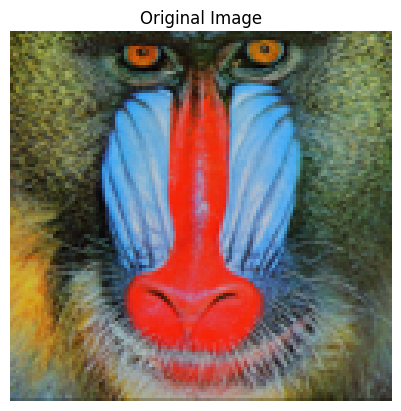``````model = hub.load(SAVED_MODEL_PATH)
``````
```Downloaded https://tfhub.dev/captain-pool/esrgan-tf2/1, Total size: 20.60MB
```
``````start = time.time()
fake_image = model(hr_image)
fake_image = tf.squeeze(fake_image)
print("Time Taken: %f" % (time.time() - start))
``````
```2021-08-14 05:57:35.821151: I tensorflow/compiler/mlir/mlir_graph_optimization_pass.cc:185] None of the MLIR Optimization Passes are enabled (registered 2)
2021-08-14 05:57:37.022074: I tensorflow/stream_executor/cuda/cuda_dnn.cc:369] Loaded cuDNN version 8100
2021-08-14 05:57:37.565251: I tensorflow/core/platform/default/subprocess.cc:304] Start cannot spawn child process: No such file or directory
Time Taken: 2.691924
```
``````# Plotting Super Resolution Image
plot_image(tf.squeeze(fake_image), title="Super Resolution")
save_image(tf.squeeze(fake_image), filename="Super Resolution")
``````
```Saved as Super Resolution.jpg
```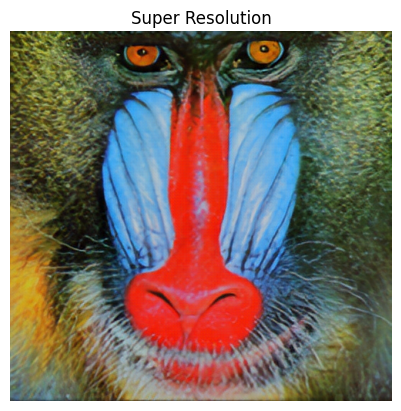### モデルのパフォーマンスの評価

``````!wget "https://lh4.googleusercontent.com/-Anmw5df4gj0/AAAAAAAAAAI/AAAAAAAAAAc/6HxU8XFLnQE/photo.jpg64" -O test.jpg
IMAGE_PATH = "test.jpg"
``````
```--2021-08-14 05:57:38--  https://lh4.googleusercontent.com/-Anmw5df4gj0/AAAAAAAAAAI/AAAAAAAAAAc/6HxU8XFLnQE/photo.jpg64
HTTP request sent, awaiting response... 200 OK
Length: 84897 (83K) [image/jpeg]
Saving to: ‘test.jpg’

test.jpg            100%[===================>]  82.91K  --.-KB/s    in 0.001s

2021-08-14 05:57:38 (108 MB/s) - ‘test.jpg’ saved [84897/84897]
```
``````# Defining helper functions
def downscale_image(image):
"""
Scales down images using bicubic downsampling.
Args:
image: 3D or 4D tensor of preprocessed image
"""
image_size = []
if len(image.shape) == 3:
image_size = [image.shape, image.shape]
else:
raise ValueError("Dimension mismatch. Can work only on single image.")

image = tf.squeeze(
tf.cast(
tf.clip_by_value(image, 0, 255), tf.uint8))

lr_image = np.asarray(
Image.fromarray(image.numpy())
.resize([image_size // 4, image_size // 4],
Image.BICUBIC))

lr_image = tf.expand_dims(lr_image, 0)
lr_image = tf.cast(lr_image, tf.float32)
return lr_image
``````
``````hr_image = preprocess_image(IMAGE_PATH)
``````
``````lr_image = downscale_image(tf.squeeze(hr_image))
``````
``````# Plotting Low Resolution Image
plot_image(tf.squeeze(lr_image), title="Low Resolution")
``````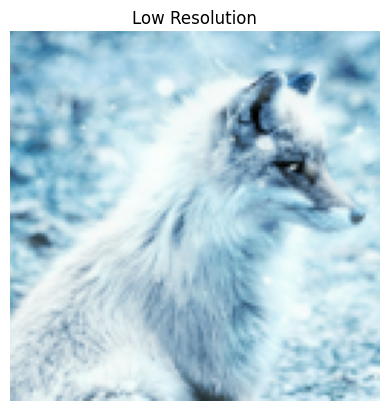``````model = hub.load(SAVED_MODEL_PATH)
``````
``````start = time.time()
fake_image = model(lr_image)
fake_image = tf.squeeze(fake_image)
print("Time Taken: %f" % (time.time() - start))
``````
```Time Taken: 1.171038
```
``````plot_image(tf.squeeze(fake_image), title="Super Resolution")
# Calculating PSNR wrt Original Image
psnr = tf.image.psnr(
tf.clip_by_value(fake_image, 0, 255),
tf.clip_by_value(hr_image, 0, 255), max_val=255)
print("PSNR Achieved: %f" % psnr)
``````
```PSNR Achieved: 28.029171
```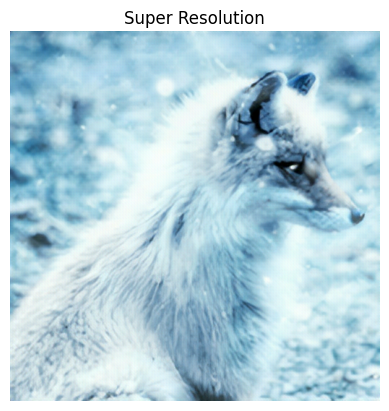``````plt.rcParams['figure.figsize'] = [15, 10]
fig, axes = plt.subplots(1, 3)
fig.tight_layout()
plt.subplot(131)
plot_image(tf.squeeze(hr_image), title="Original")
plt.subplot(132)
fig.tight_layout()
plot_image(tf.squeeze(lr_image), "x4 Bicubic")
plt.subplot(133)
fig.tight_layout()
plot_image(tf.squeeze(fake_image), "Super Resolution")
plt.savefig("ESRGAN_DIV2K.jpg", bbox_inches="tight")
print("PSNR: %f" % psnr)
``````
```PSNR: 28.029171
```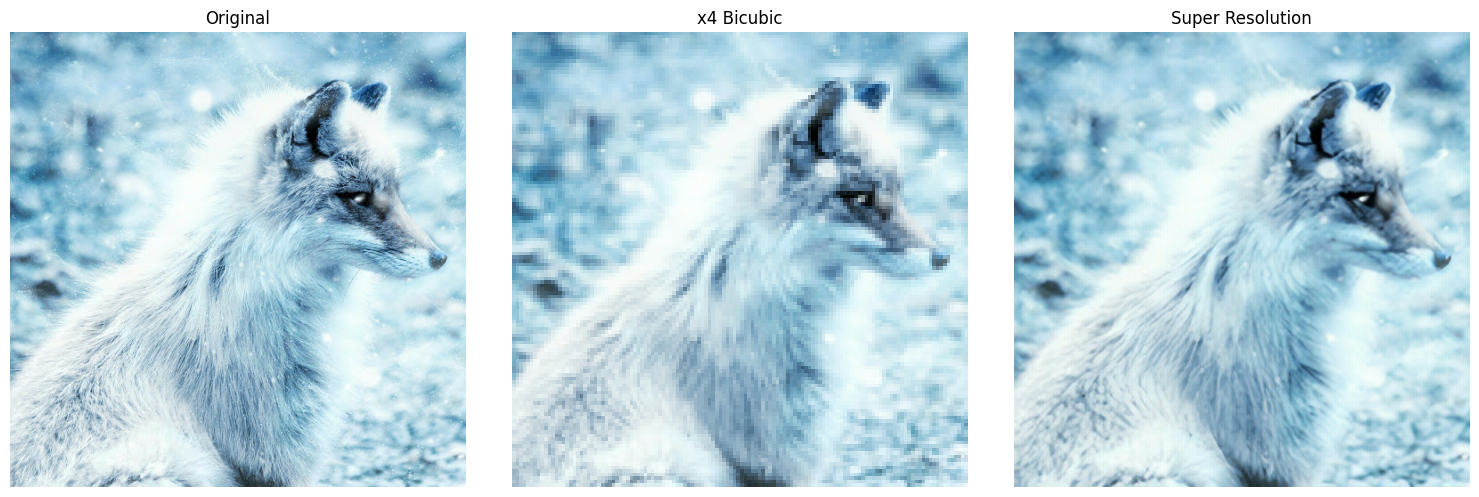[{ "type": "thumb-down", "id": "missingTheInformationINeed", "label":"必要な情報がない" },{ "type": "thumb-down", "id": "tooComplicatedTooManySteps", "label":"複雑すぎる / 手順が多すぎる" },{ "type": "thumb-down", "id": "outOfDate", "label":"最新ではない" },{ "type": "thumb-down", "id": "translationIssue", "label":"翻訳に関する問題" },{ "type": "thumb-down", "id": "samplesCodeIssue", "label":"Samples / code issue" },{ "type": "thumb-down", "id": "otherDown", "label":"その他" }]
[{ "type": "thumb-up", "id": "easyToUnderstand", "label":"わかりやすい" },{ "type": "thumb-up", "id": "solvedMyProblem", "label":"問題の解決に役立った" },{ "type": "thumb-up", "id": "otherUp", "label":"その他" }]